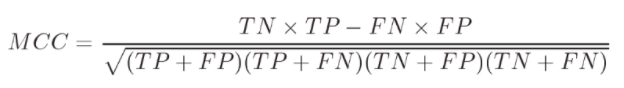# Matthews Correlation Coefficient

The Matthews correlation coefficient (MCC), invented by Brian Matthews in 1975, is a tool for model evaluation. It measures the differences between actual values and predicted values and is equivalent to the chi-square statistic for a 2 x 2 contingency table (Kaden et al., 2014).

The coefficient takes into account true negatives, true positives, false negatives and false positives. This reliable measure produces high scores only if the prediction returns good rates for all four of these categories (Chicco & Jurman, 2020).

## Matthews Correlation Coefficient Formula

The formula is calculated with the equation (Măndoiu, 2007):Like most correlation coefficients, MCC ranges between -1 and 1, where (Vothihong et al., 2017):

• 1 is the best agreement between actuals and predictions,
• zero is no agreement at all. In other words, the prediction is random with respect to actuals.

In some contexts, such as secondary structure prediction in bioinformatics, MCC is equivalent to Pearson’s Correlation Coefficient (Baldi et al., 2000).

## References

Baldi, P. et al. (2000). Assessing the Accuracy of Prediction Algorithms for Classifi cation: An Overview. Bioinformatics, Volume 16, Issue 5, May, Pages 412–424, https://doi.org/10.1093/bioinformatics/16.5.412
Chicco, D. & Jurman, G. (2020). The advantages of the Matthews correlation coefficient (MCC) over F1 score and accuracy in binary classification evaluation. BMC Genomics volume 21, Article number: 6.
Kaden, M. et al., (2014). Optimization of General Statistical Accuracy Measures for Classification Based on Learning VectorQuantization. ESANN 2014 proceedings, European Symposium on Artificial Neural Networks, Computational Intelligence
and Machine Learning. Bruges (Belgium), 23-25 April 2014, i6doc.com publ., ISBN 978-287419095-7.
Available from http://www.i6doc.com/fr/livre/?GCOI=28001100432440.
Vothihong, P. et al. (2007). Python: End-to-end Data Analysis. Packt Publishing.
Yang, Z. (2007). Predicting Palmitoylation Sites Using a Regularised Bio-basis Function Neural Network. Bioinformatics Research and Applications
Third International Symposium, ISBRA 2007, Atlanta, GA, USA, May 7-10, 2007, Proceedings. Springer.

CITE THIS AS:
Stephanie Glen. "Matthews Correlation Coefficient" From StatisticsHowTo.com: Elementary Statistics for the rest of us! https://www.statisticshowto.com/matthews-correlation-coefficient/
---------------------------------------------------------------------------Need help with a homework or test question? With Chegg Study, you can get step-by-step solutions to your questions from an expert in the field. Your first 30 minutes with a Chegg tutor is free!